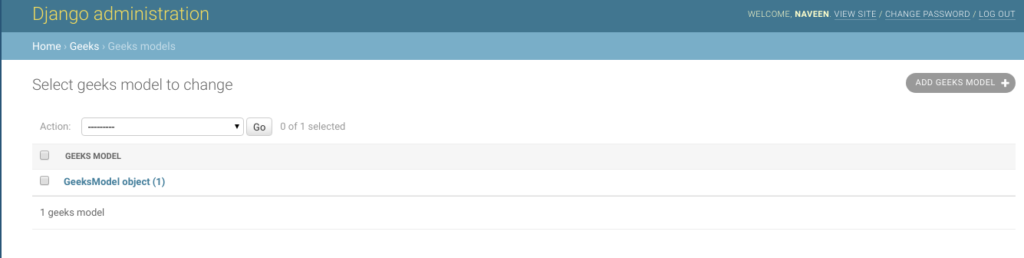# TimeField – Django Models

TimeField is a time field which stores time, represented in Python by a `datetime.time` instance. As the name suggests, this field is used to store an object of datetime created in python. The default form widget for this field is a `TextInput`. The admin uses two separate `TextInput` widgets with JavaScript shortcuts.

Syntax

`field_name = models.TimeField(auto_now=False, auto_now_add=False, **options)`
• #### TimeField.auto_now

Automatically set the field to now every time the object is saved. Useful for “last-modified” timestamps. Note that the current time is always used; it’s not just a default value that you can override.
The field is only automatically updated when calling Model.save(). The field isn’t updated when making updates to other fields in other ways such as QuerySet.update(), though you can specify a custom value for the field in an update like that.

Automatically set the field to now when the object is first created. Useful for creation of timestamps. Note that the current time is always used; it’s not just a default value that you can override. So even if you set a value for this field when creating the object, it will be ignored. If you want to be able to modify this field, set the following instead of auto_now_add=True:

• For TimeField: default=datetime.time.now – from `datetime.now()`
• For TimeField: default=timezone.now – from `django.utils.timezone.now()`

Note: The options auto_now_add, auto_now, and default are mutually exclusive. Any combination of these options will result in an error.

## Django Model TimeField Explanation

Illustration of TimeField using an Example. Consider a project named `geeksforgeeks` having an app named `geeks`.

Refer to the following articles to check how to create a project and an app in Django.

Enter the following code into `models.py` file of geeks app.

 `from` `django.db ``import` `models ` `from` `django.db.models ``import` `Model ` `# Create your models here. ` ` `  `class` `GeeksModel(Model): ` `    ``geeks_field ``=` `models.TimeField() `

Add the geeks app to `INSTALLED_APPS`

 `# Application definition ` ` `  `INSTALLED_APPS ``=` `[ ` `    ``'django.contrib.admin'``, ` `    ``'django.contrib.auth'``, ` `    ``'django.contrib.contenttypes'``, ` `    ``'django.contrib.sessions'``, ` `    ``'django.contrib.messages'``, ` `    ``'django.contrib.staticfiles'``, ` `    ``'geeks'``, ` `] `

Now when we run `makemigrations` command from the terminal,

`Python manage.py makemigrations`

A new folder named migrations would be created in `geeks` directory with a file named `0001_initial.py`

 `# Generated by Django 2.2.5 on 2019-09-25 06:00 ` ` `  `from` `django.db ``import` `migrations, models ` ` `  `class` `Migration(migrations.Migration): ` ` `  `    ``initial ``=` `True` ` `  `    ``dependencies ``=` `[ ` `    ``] ` ` `  `    ``operations ``=` `[ ` `        ``migrations.CreateModel( ` `            ``name ``=``'GeeksModel'``, ` `            ``fields ``=``[ ` `                ``(``'id'``,  ` `                  ``models.AutoField( ` `                  ``auto_created ``=` `True``, ` `                  ``primary_key ``=` `True``, ` `                  ``serialize ``=` `False``,  ` `                  ``verbose_name ``=``'ID'` `                ``)), ` `                ``(``'geeks_field'``, models.TimeField()), ` `            ``], ` `        ``), ` `    ``] `

Now run,

`Python manage.py migrate`

Thus, an` geeks_field `TimeField is created when you run migrations on the project. It is a field to store `datetime.time` python object.

## How to use TimeField ?

TimeField is used for storing python datetime.time instance in the database. One can store any type of time using the same in the database. Let’s try storing a date in model created above.

 `# importing the model ` `# from geeks app ` `from` `geeks.models ``import` `GeeksModel ` ` `  `# importing datetime module ` `import` `datetime ` ` `  `# creating an instance of  ` `# datetime.time ` `# time(hour = 0, minute = 0, second = 0) ` `d ``=` `datetime.time(``10``, ``33``, ``45``) ` ` `  `# creating an instance of  ` `# GeeksModel ` `geek_object ``=` `GeeksModel.objects.create(geeks_field ``=` `d) ` `geek_object.save() `

Now let’s check it in admin server. We have created an instance of GeeksModel.## Field Options

Field Options are the arguments given to each field for applying some constraint or imparting a particular characteristic to a particular Field. For example, adding an argument `null = True` to TimeField will enable it to store empty values for that table in relational database.
Here are the field options and attributes that an TimeField can use.

Field Options Description
Null If True, Django will store empty values as NULL in the database. Default is False.
Blank If True, the field is allowed to be blank. Default is False.
Choices An iterable (e.g., a list or tuple) of 2-tuples to use as choices for this field.
Default The default value for the field. This can be a value or a callable object. If callable it will be called every time a new object is created.
help_text Extra “help” text to be displayed with the form widget. It’s useful for documentation even if your field isn’t used on a form.
primary_key If True, this field is the primary key for the model.
Unique If True, this field must be unique throughout the table.

My Personal Notes arrow_drop_upCheck out this Author's contributed articles.

If you like GeeksforGeeks and would like to contribute, you can also write an article using contribute.geeksforgeeks.org or mail your article to contribute@geeksforgeeks.org. See your article appearing on the GeeksforGeeks main page and help other Geeks.

Please Improve this article if you find anything incorrect by clicking on the "Improve Article" button below.

Article Tags :

Be the First to upvote.

Please write to us at contribute@geeksforgeeks.org to report any issue with the above content.